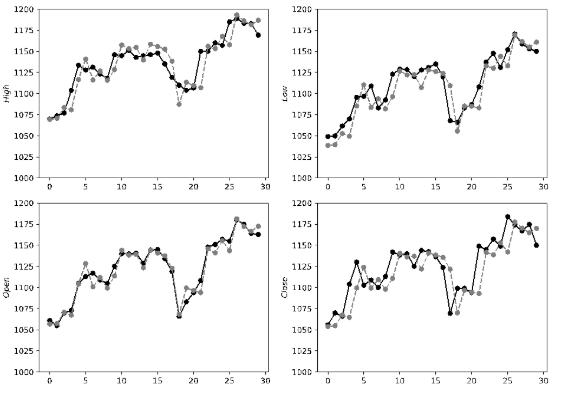# 我学会了用 Python 预测股票价格

0收藏

1 var hqstrsh601006="大秦铁路,6.980,6.960,7.010,7.070,6.950,7.010,7.020,121033256,847861533.000,18900, 7.010,214867,7.000,66500,6.990,386166,6.980,336728,6.970,273750,7.020,836066,7.030,630800,7.040,936306,7.050,579400,7.060,2016-03-18,15:00:00,00";

``````
0：<大秦铁路>，股票名字
1：<< span="">6.980>，今日开盘价
2：<< span="">6.960>，昨日收盘价
3：<< span="">7.010>，当前价格
4：<< span="">7.070>，今日最高价
5：<< span="">6.950>，今日最低价
6：<< span="">7.010>，竞买价，即“买一”报价
7：<< span="">7.020>，竞卖价，即“卖一”报价
8：<< span="">121033256>，成交的股票数，由于股票交易以一百股为基本单位，所以在使用时，通常把该值除以一百
9：<< span="">847861533.000>，成交金额，单位为“元”，为了一目了然，通常以“万元”为成交金额的单位，所以通常把该

10：<< span="">18900>，“买一”申请4695股，即47手
11：<< span="">7.010>，“买一”报价
12：<< span="">214867>，“买二”
13：<< span="">7.000>，“买二”
14：<< span="">66500>，“买三”
15：<< span="">6.990>，“买三”
16：<< span="">386166>，“买四”
17：<< span="">6.980>，“买四”
18：<< span="">336728>，“买五”
19：<< span="">6.970>，“买五”
20：<< span="">273750>，“卖一”申报3100股，即31手
21：<< span="">7.020>，“卖一”报价
(22,23),(24,25),(26,27),(28,29)分别为“卖二”至“卖四的情况”
：<< span="">2016-03-18>，日期
：<< span="">15:00:00>，时间``````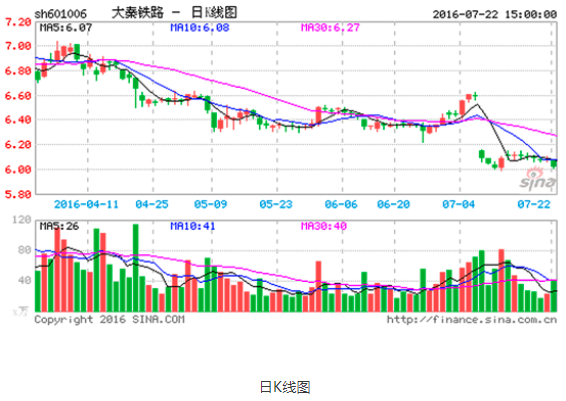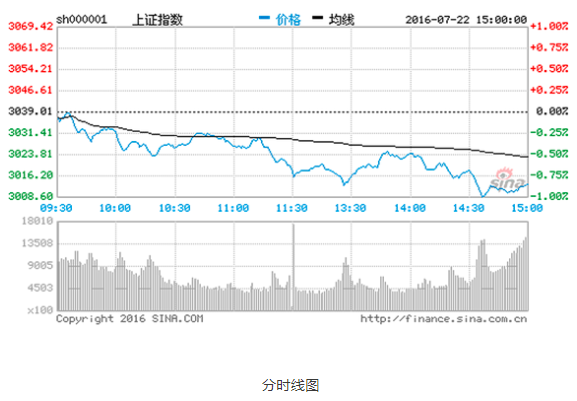``````import pandas as pd
import datetime as dt
High         Low        Open       Close    Volume   Adj Close
# Date
# 2019-08-01    977.000000  953.020020  976.51001   959.299988  3508952 959.299988
# 2019-08-02    957.979980  943.000000  944.00000   954.450012  3971940 954.450012
# 2019-08-05    954.000000  940.000000  945.00000   942.429993  3677431 942.429993
# 2019-08-06    948.000000  923.799988  931.00000   946.299988  4399116 946.299988
# 2019-08-07    955.530029  945.000000  949.50000   945.000000  2686998 945.000000

kldata=data.values[:,[2,3,1,0]] # 分别对应开盘价、收盘价、最低价和最高价
from pyecharts import options as opts
from pyecharts.charts import Kline

yaxis_opts=opts.AxisOpts(is_scale=True),
xaxis_opts=opts.AxisOpts(is_scale=True),
title_opts=opts.TitleOpts(title=""))
kobj.render()``````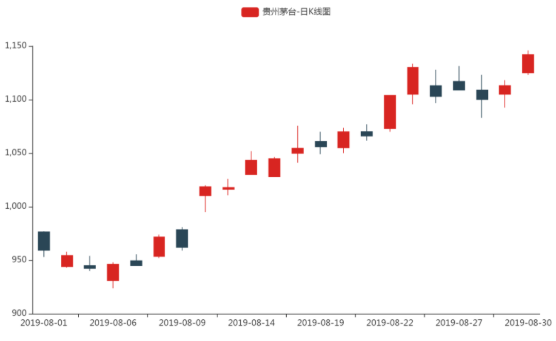VAR模型类似联立方程，将多个变量包含在一个统一的模型中，共同利用多个变量信息，比起仅使用单一时间序列的ARIMA等模型，其涵盖的信息更加丰富，能更好地模拟现实经济体，因而用于预测时能够提供更加贴近现实的预测值。此处拟基于贵州茅台股票数据，建立VAR的预测模型。使用后30天的数据作为验证集，剩余的数据用于建立预测模型。本节从VAR模型的平稳性检验出发，依次完成VAR模型的定阶及建模预测，最终通过分析验证集上的准确率来评估预测效果。

`````` import statsmodels.tsa.stattools as stat
import datetime as dt
import pandas as pd
import numpy as np

subdata = data.iloc[:-30,:4]
for i in range(4):
print("指标 ",data.columns[i]," 单位根检验的p值为：",pvalue)
# 指标  High  单位根检验的p值为：0.9955202280850401
# 指标  Low  单位根检验的p值为：0.9942509439755689
# 指标  Open  单位根检验的p值为：0.9938548193990323
# 指标  Close  单位根检验的p值为：0.9950049124079876``````

``````subdata_diff1 = subdata.iloc[1:,:].values - subdata.iloc[:-1,:].values
for i in range(4):
print("指标 ",data.columns[i]," 单位根检验的p值为：",pvalue)
# 指标  High  单位根检验的p值为：0.0
# 指标  Low  单位根检验的p值为：0.0
# 指标  Open  单位根检验的p值为：0.0
# 指标  Close  单位根检验的p值为：0.0``````

VAR模型定阶

``````# 模型阶数从1开始逐一增加
rows, cols = subdata_diff1.shape
aicList = []
lmList = []

for p in range(1,11):
baseData = None
for i in range(p,rows):
tmp_list = list(subdata_diff1[i,:]) + list(subdata_diff1[i-p:i].flatten())
if baseData is None:
baseData = [tmp_list]
else:
baseData = np.r_[baseData, [tmp_list]]
X = np.c_[*baseData.shape,baseData[:,cols:]]
Y = baseData[:,0:cols]
coefMatrix = np.matmul(np.matmul(np.linalg.inv(np.matmul(X.T,X)),X.T),Y)
aic = np.log(np.linalg.det(np.cov(Y - np.matmul(X,coefMatrix),rowvar=False))) + 2*(coefMatrix.shape-1)**2*p/baseData.shape
aicList.append(aic)
lmList.append(coefMatrix)

#对比查看阶数和AIC
pd.DataFrame({"P":range(1,11),"AIC":aicList})
#   P   AIC
# 0 1   13.580156
# 1 2   13.312225
# 2 3   13.543633
# 3 4   14.266087
# 4 5   15.512437
# 5 6   17.539047
# 6 7   20.457337
# 7 8   24.385459
# 8 9   29.438091
# 9 10  35.785909``````

``````p = np.argmin(aicList)+1
n = rows
preddf = None
for i in range(30):
predData = list(subdata_diff1[n+i-p:n+i].flatten())
predVals = np.matmul(+predData,lmList[p-1])
# 使用逆差分运算，还原预测值
predVals=data.iloc[n+i,:].values[:4]+predVals
if preddf is None:
preddf = [predVals]
else:
preddf = np.r_[preddf, [predVals]]
# 为subdata_diff1增加一条新记录
subdata_diff1 = np.r_[subdata_diff1, [data.iloc[n+i+1,:].values[:4] - data.iloc[n+i,:].values[:4]]]

#分析预测残差情况
(np.abs(preddf - data.iloc[-30:data.shape,:4])/data.iloc[-30:data.shape,:4]).describe()
#       High         Low         Open       Close
# count 30.000000   30.000000   30.000000   30.000000
# mean  0.010060    0.009380    0.005661    0.013739
# std   0.008562    0.009968    0.006515    0.013674
# min   0.001458    0.000115    0.000114    0.000130
# 25%   0.004146    0.001950    0.001653    0.002785
# 50%   0.007166    0.007118    0.002913    0.010414
# 75%   0.014652    0.012999    0.006933    0.022305
# max   0.039191    0.045802    0.024576    0.052800``````

``````import matplotlib.pyplot as plt
plt.figure(figsize=(10,7))
for i in range(4):
plt.subplot(2,2,i+1)
plt.plot(range(30),data.iloc[-30:data.shape,i].values,'o-',c='black')
plt.plot(range(30),preddf[:,i],'o--',c='gray')
plt.ylim(1000,1200)
plt.ylabel("\$"+data.columns[i]+"\$")
plt.show()
v = 100*(1 - np.sum(np.abs(preddf - data.iloc[-30:data.shape,:4]).values)/np.sum(data.iloc[-30:data.shape,:4].values))
print("Evaluation on test data: accuracy = %0.2f%% \n" % v)
# Evaluation on test data: accuracy = 99.03%``````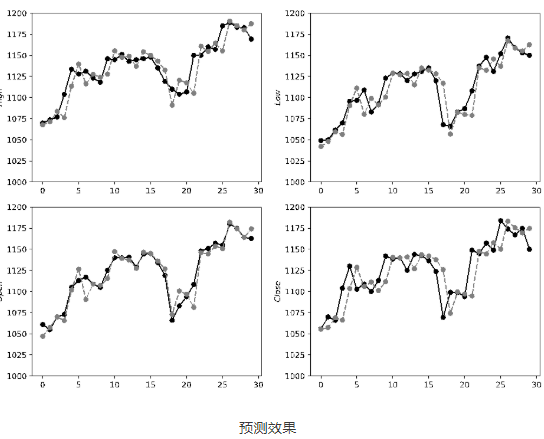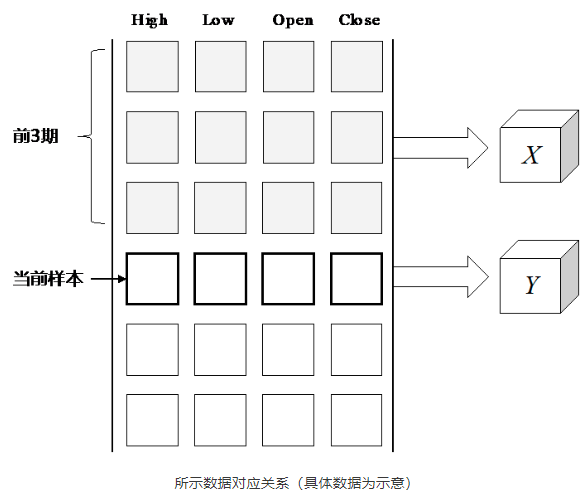`````` 1SEQLEN = 21
dim_in = 4
dim_out = 4
pred_len = 30
vmean = data.iloc[:,:4].apply(lambda x:np.mean(x))
vstd = data.iloc[:,:4].apply(lambda x:np.std(x))
t0 = data.iloc[:,:4].apply(lambda x:(x-np.mean(x))/np.std(x)).values
X_train = np.zeros((t0.shape-SEQLEN-pred_len, SEQLEN, dim_in))
Y_train = np.zeros((t0.shape-SEQLEN-pred_len, dim_out),)
X_test = np.zeros((pred_len, SEQLEN, dim_in))
Y_test = np.zeros((pred_len, dim_out),)
for i in range(SEQLEN, t0.shape-pred_len):
Y_train[i-SEQLEN] = t0[i]
X_train[i-SEQLEN] = t0[(i-SEQLEN):i]
for i in range(t0.shape-pred_len,t0.shape):
Y_test[i-t0.shape+pred_len] = t0[i]
X_test[i-t0.shape+pred_len] = t0[(i-SEQLEN):i]``````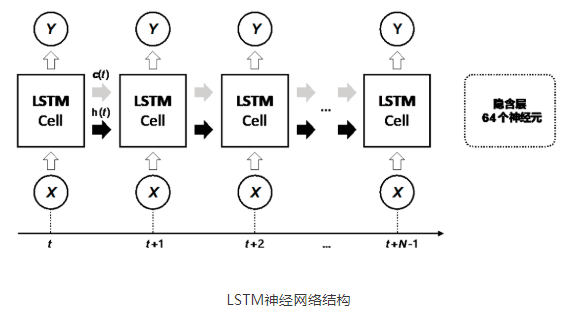``````from keras.layers import LSTM, Dense
from keras.models import Sequential
model = Sequential()
model.compile(loss = 'mean_squared_error', optimizer = 'rmsprop')
history = model.fit(X_train, Y_train, epochs=200, batch_size=10, validation_split=0)
# Epoch 1/200
# 1350/1350 [==============================] - 1s 1ms/step - loss: 0.0447
# Epoch 2/200
# 1350/1350 [==============================] - 1s 737us/step - loss: 0.0059
# Epoch 3/200
# 1350/1350 [==============================] - 1s 743us/step - loss: 0.0043
# ......
# Epoch 200/200
# 1350/1350 [==============================] - 1s 821us/step - loss: 9.2794e-04``````

``preddf=model.predict(X_test)*vstd.values+vmean.values``

``````preddf
# array([[1069.35781887, 1038.57915742, 1056.77147186, 1053.83827734],
#       [1070.65142282, 1039.58533719, 1057.34561875, 1054.85567074],
#       [1083.58529328, 1052.70457308, 1070.78824637, 1067.49741882],
#
#       [1186.19297789, 1161.52758381, 1172.33666591, 1170.44623263],
#       [1181.42680223, 1155.14778501, 1166.5726204 , 1165.00336968],
#       [1186.75600881, 1160.84733425, 1172.37636963, 1170.09819923]])

preddf.shape
# (30, 4)``````

``````import matplotlib.pyplot as plt
plt.figure(figsize=(10,7))
for i in range(4):
plt.subplot(2,2,i+1)
plt.plot(range(30),data.iloc[-30:data.shape,i].values,'o-',c='black')
plt.plot(range(30),preddf[:,i],'o--',c='gray')
plt.ylim(1000,1200)
plt.ylabel("\$"+data.columns[i]+"\$")
plt.show()
v = 100*(1 - np.sum(np.abs(preddf - data.iloc[-30:data.shape,:4]).values)/np.sum (data.iloc[-30:data.shape,: 4].values))
print("Evaluation on test data: accuracy = %0.2f%% \n" % v)
# Evaluation on test data: accuracy = 99.01%``````